# Introduction to R:loa

## R:loa

This note provides an brief background and overview introduction to the R package loa.

The lattice1 package that comes with R draws graphs.

loa is short for Lattice Optional extras and Add-ins, and that pretty much describes loa.

This note assumes you already have R. If not but the note is of interest, R is open-source and free-to-distribute, and you can get it from >The R Project.

## Background

I originally packaged loa because I had a lot of plot code, most modifications of or extensions to existing lattice code, that I was using and replicating in several projects and I wanted to rationalise things.

The intention was also to automate and simplify some of the data handling needed when lattice plotting2.

## Getting loa

Installing loa from CRAN, the main R package archive:

#In R, type:
install.packages("loa")

Installing the developers version:

install.packages("loa", repos="http://R-Forge.R-project.org")

To load loa once it is installed:

library(loa) #or require(loa)

## The loa Inventory

loa version 0.2.47.13 contains:

• Several plot functions, e.g. loaPlot(), RgoogleMapsPlot() and trianglePlot();
• Add-ins like panel functions to change plot outputs; and
• Some functions to automate handling of some of the more mundane plot parameters.

loa also contains one data set, lat.lon.meuse, and one map, roadmap.meuse, which are used in examples in the loa documentation.

## The Datasets

lat.lon.meuse is a data.set containing metal concentrations measured in soil and sediment samples collected from a section of the Meuse floodplain in the Netherlands.

It is a modification of the meuse data.set in the sp package.4 It contains concentrations of four metals (cadmium, copper, lead and zinc), sampling location (latitude, longitude, elevation), and other related information (e.g. soil type) for 155 locations.

There are further details on both in the package documentation, see ?lat.lon.meuse or ?roadmap.meuse.

## Working with loa

loa uses lattice plotting strategies5.

Plot dimensions are set using a formula as the first argument of the plot command.

For those unfamiliar with the approach, it is probably easiest to think of a lattice plot as a model like lm() but with a formula that maps one term onto a series of axes rather than a series of inputs.

For example, loaPlot() uses a formula structure z ~ x * y | cond, where:

• x and y are the x and y axes,

• z is an optional term controlling the appearance (color and size) of points plotted at (x, y), and

• cond, also optional, is any additional term that can be used to subsample (x,y,z) data when plotting subsets separately.

The formula terms may be supplied directly or taken from a source identified using a second optional plot argument data. If supplied, data should be a data.frame or something that can be converted into one using as.data.frame(data), e.g.:

loaPlot(cadmium~longitude*latitude, data=lat.lon.meuse)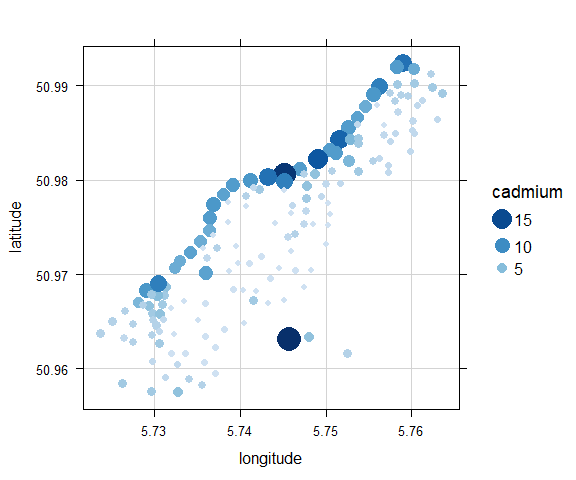Here, the z term, the cadmium data-series, is used to both color and size points plotted at x (longitude) and y (latitude) locations.

Additional arguments may be used to fine-tune the appearance of the plot, e.g. groups to group data within the plot:

loaPlot(cadmium~longitude*latitude, data=lat.lon.meuse,
groups=lat.lon.meuse\$soil)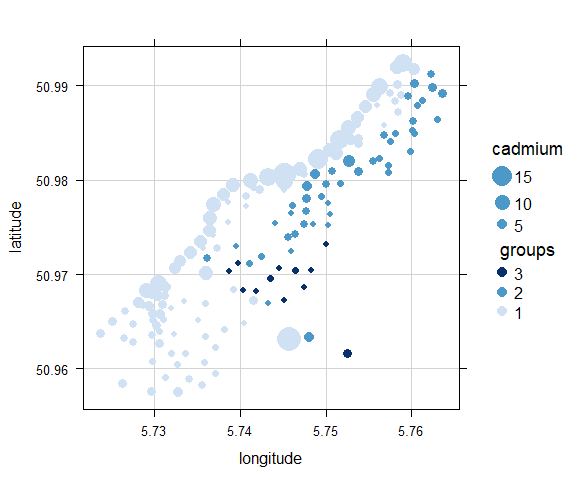Here, the plot color-codes the points according to soil (soil type 1-3), also in lat.lon.meuse.

Note: By default, groups supersedes z as the coloring term when set but the points remain size scaled according to z.

By comparison, conditioning by soil type would produce:

loaPlot(cadmium~longitude*latitude|soil, data=lat.lon.meuse,
layout=c(3,1))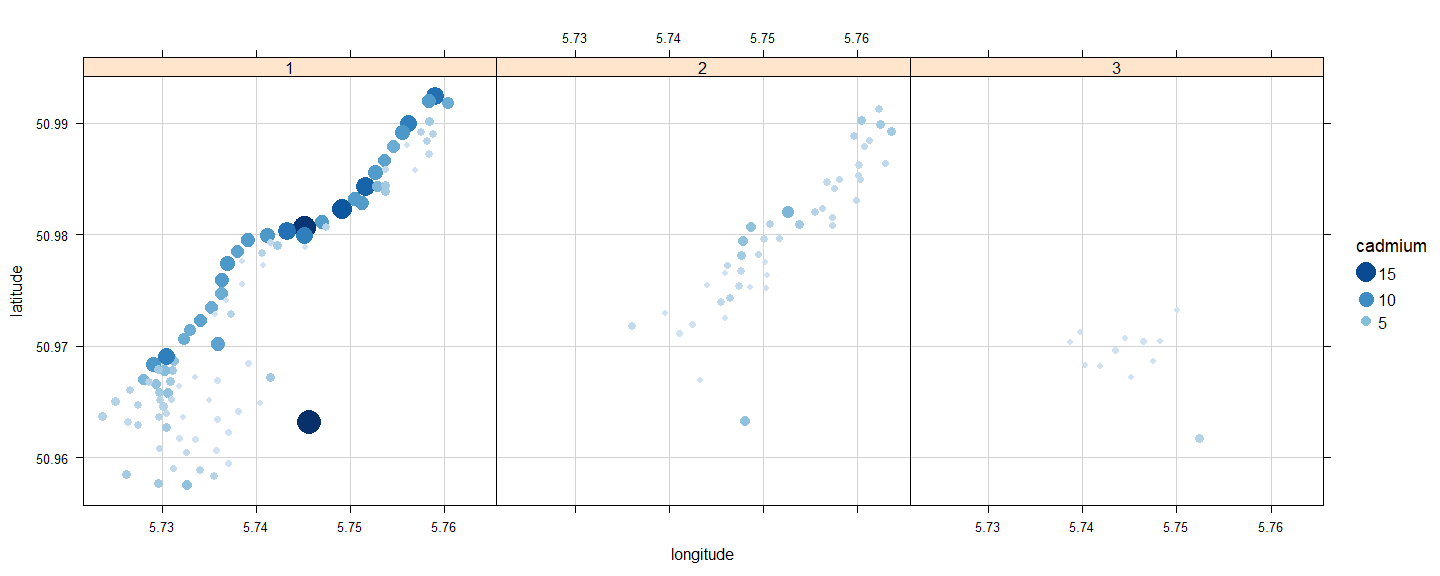This plots data of different soil types in discrete plot panels, each, by default, plotted using the same x, y, z and groups plot settings. The standard lattice argument layout is also used to plot the lattice sub-plots (or panels) in a row.

## loaPlot

Already introduced above, loaPlot() is a z ~ x * y bubble plot with default color (col) and size (cex) scaling linked to the z term unless groups are set, in which case cex is linked to z and col is linked to groups.

Many of the additional plot arguments are the same as in lattice, but some options have been enhanced or simplified, e.g.:

#fixed col (but automatic z size scale)
#key scale manually extended
col="darkred", key.z.at=c(3,6,9,12,15,18))
#replacing default blue scale with RColorBrewer palette
#use color scale key like levelplot
col.regions="Spectral", alpha=0.5,
key.fun=draw.loaColorKey)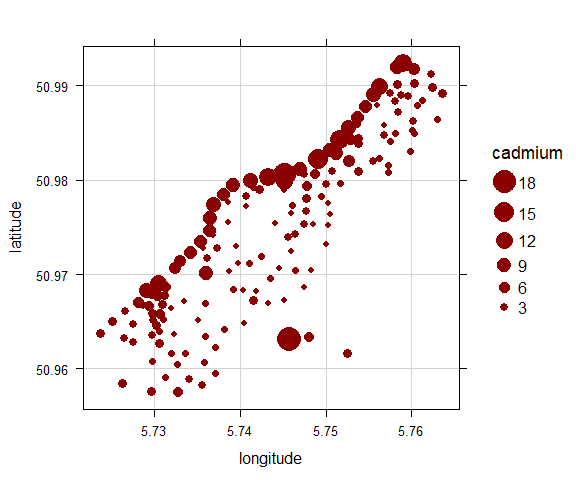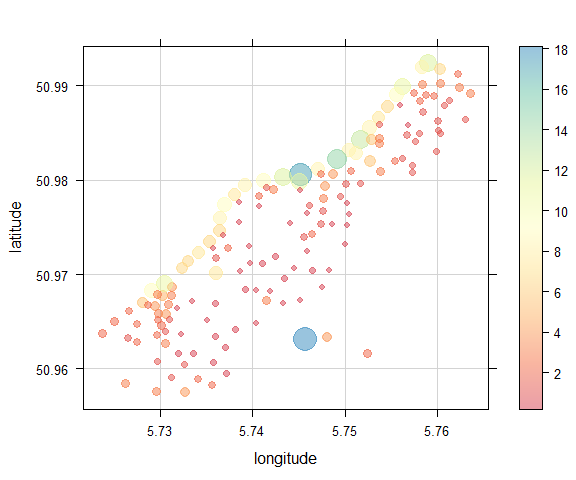• As in lattice, col sets point color directly and col.regions sets the color palette colors are selected from. But loa also uses RColorBrewer6 to handle color ranges. So, the loa version of col.regions is a little more flexible than in lattice. For example, loaPlot() handles arguments like col.regions = c(“green”, “red”).

• unlike xyplot() in lattice, loaPlot() automatically generates a plot key if it thinks you need one, but (like in lattice), key and key… arguments can be used to modify the key, e.g. key = FALSE to remove the key.

• I have tried to simplify some common arguments that use list structures in lattice. So, e.g. instead of using key=list(z=list(at=c(…))) you can use key.z.at=c(…) to manually set the points shown in the key. There are trade-offs in doing this, so it is currently only applied to selected cases in loaPlot(), but it does mean you can more easily change grid appearance with e.g. grid.col=2 or grid.lty=3, etc.

• I also linked the loaPlot() argument zlab to key.z.main so you could rename the z key like you would the x and y axes.

Note: The loaPlot() key can include z and groups elements. So, you need to specify which you want to change, hence key.z.at=… (not key.at=…).

Like lattice plots, loaPlot() also accepts other panel arguments to produce other plot types.

For example:

#panel.binPlot
panel=panel.binPlot, breaks=20, statistic=max)
#panel.surfaceSmooth
panel=panel.surfaceSmooth, too.far=0.1)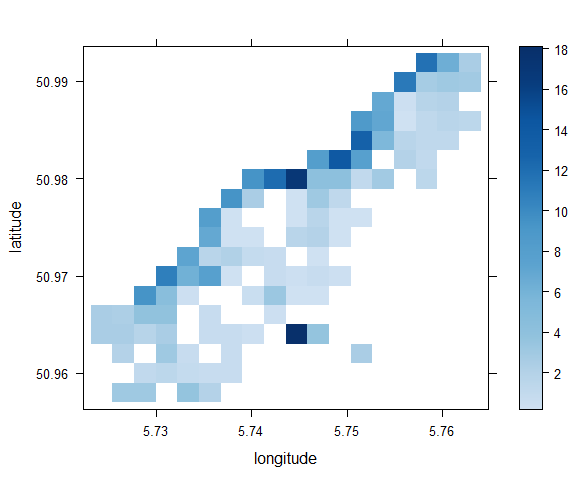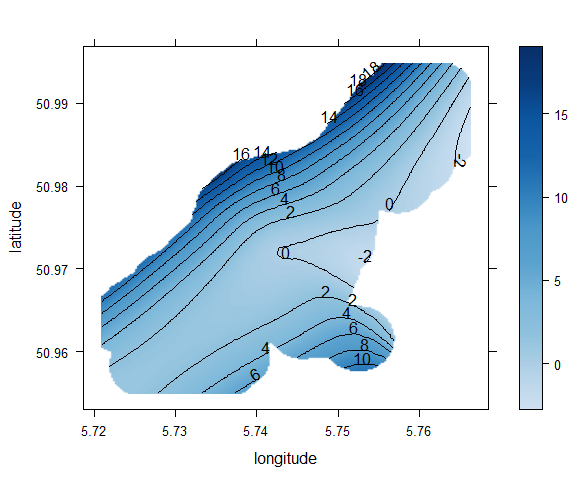• The binPlot panel expects x, y and z inputs and generates a heat map and color key similar to levelplot() but without the extra coding needed to get from xyplot() to levelplot() inputs. By default, it calculates means for each bin if z is supplied or generates a warning and counts bin sizes if not. You can also use arguments like breaks and statistic to change the number of bins and the way the binned data is handled.
• The surfaceSmooth panel also expects x, y and z and fits a color-surface to (x, y, z). By default, it extrapolates the surface to the x and y plot limits. However, the too.far argument (based on too.far in GAM visualizations in the mgcv package7) can to used to limit the amount of extrapolation (0.1 = 10% of plot range from nearest point).

loaPlot() also allows you to supply multiple z values in form z1 + z2 + … + zn ~ x + y.

Note: This option is not intended to be used with loaPlot() without additional option settings because it would just create over-plotting at each (x, y) point. However, it is useful when used with either z case panelling or specialist panels designed for use with mulitple z inputs, e.g.:

#panel.zcases to condition by z1, z2, etc
data=lat.lon.meuse, zlab="Metals",
panel.zcases = TRUE)
#using the panel.zcasePiePlot and z1, z2, etc
data=lat.lon.meuse, zcaselab="Metals",
panel=panel.zcasePiePlot)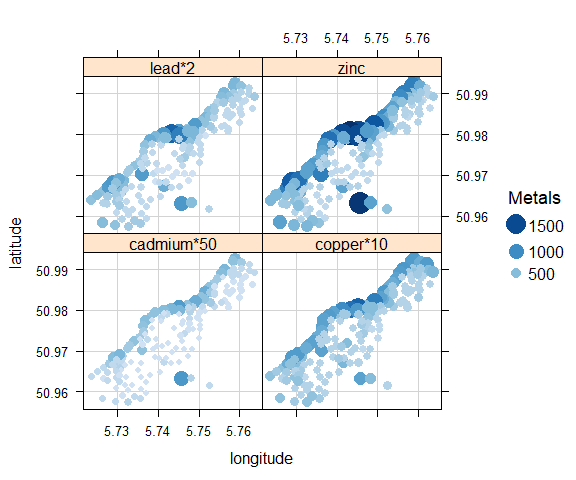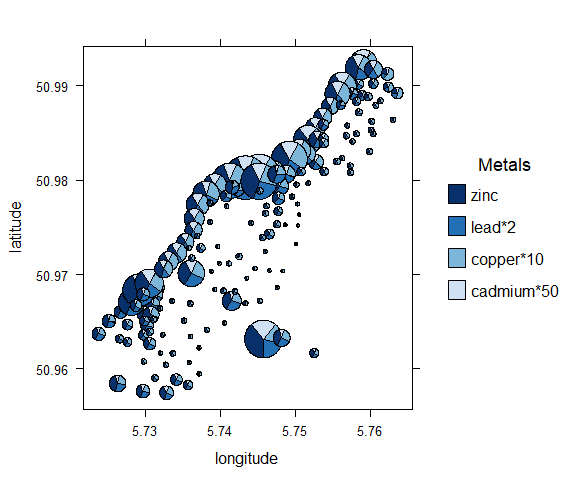For further information on loaPlot(), see in-package documentation ?loaPlot.

## Other Plots

Other plots in loa include RgoogleMapsPlot(), trianglePlot(), stackPlot() and loaBarPlot():

#RgoogleMapsPlot
#trianglePlot
#stackPlot
#loaBarPlot
loaBarPlot(copper~soil, data=lat.lon.meuse, stat=mean)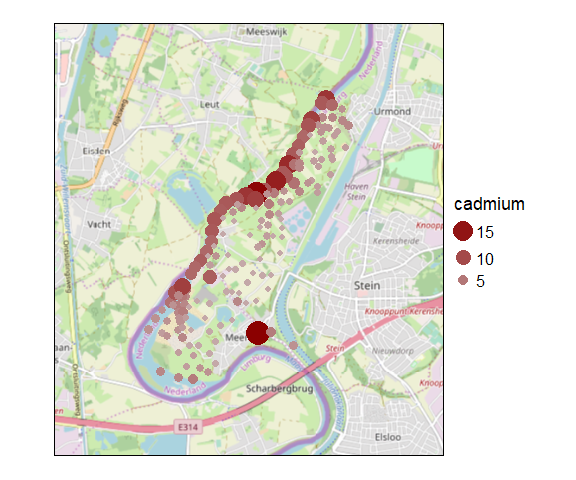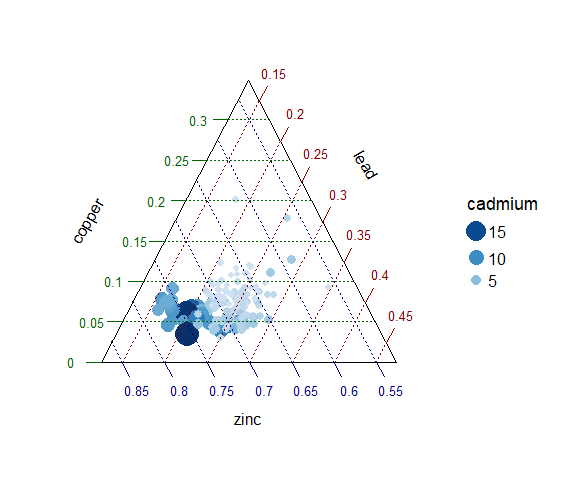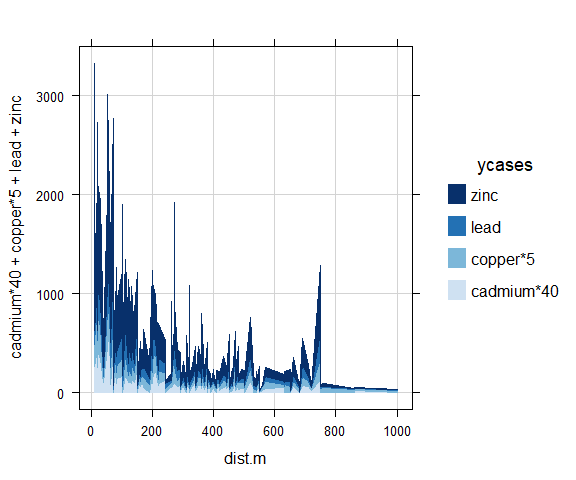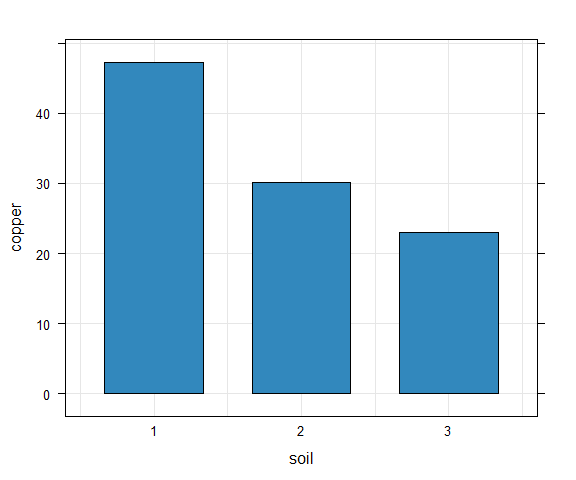• RgoogleMapsPlot() is an extension of loaPlot() that adds a map layer to plots using the RgoogleMaps package8. It uses a z ~ latitude * longitude | cond plot structure and accepts loaPlot() arguments to modify data layers as well as additional arguments to modify the map layer.
• trianglePlot() is a triangle (or ternary) plot that uses (a,b,c) axes and a z ~ a + b + c | cond plot structure.
• stackPlot() is a simple data-series stacking plot that uses a y1 + y2 + … yn ~ x | cond plot structure.
• loaBarPlot() is a simple bar plot that currently uses either a y~x|cond and groups plot structure or a more conventional x, y, groups, cond plot structure.

For further information on these, see in-package documentation.

Any thoughts, suggestions or problems, please let me know > Karl

1. Sarkar D (2008). Lattice: Multivariate Data Visualization with R. Springer, New York. ISBN 978-0-387-75968-5, <URL: http://lmdvr.r-forge.r-project.org>.↩︎

2. I presented earlier thoughts on this topic in e.g. Ropkins, K., Carslaw, D.C., Munir, S., Chen, H. R. (2012) lattice and RgoogleMaps: A practical framework for the development of new geovisualizations. ASA 2012 JSM. San Diego, US.↩︎

3. Code handling and outputs may vary with package version.↩︎

4. Pebesma EJ, Bivand RS (2005). “Classes and methods for spatial data in R.” R News, 5(2), 9-13. <URL: https://CRAN.R-project.org/doc/Rnews/>., Bivand RS, Pebesma E, Gomez-Rubio V (2013). Applied spatial data analysis with R, Second edition. Springer, NY. <URL: https://asdar-book.org/>.↩︎

5. This is described in early chapters of Sarkar D (2008). Lattice: Multivariate Data Visualization with R. Springer, New York. ISBN 978-0-387-75968-5, <URL: http://lmdvr.r-forge.r-project.org>.↩︎

6. Neuwirth E (2014). RColorBrewer: ColorBrewer Palettes. R package version 1.1-2, <URL: https://CRAN.R-project.org/package=RColorBrewer>.↩︎

7. Wood SN (2011). “Fast stable restricted maximum likelihood and marginal likelihood estimation of semiparametric generalized linear models.” Journal of the Royal Statistical Society (B), 73(1), 3-36., Wood S, N., Pya, S“afken B (2016).”Smoothing parameter and model selection for general smooth models (with discussion)." Journal of the American Statistical Association, 111, 1548-1575., Wood SN (2004). “Stable and efficient multiple smoothing parameter estimation for generalized additive models.” Journal of the American Statistical Association, 99(467), 673-686., Wood S (2017). Generalized Additive Models: An Introduction with R, 2 edition. Chapman and Hall/CRC., Wood SN (2003). “Thin-plate regression splines.” Journal of the Royal Statistical Society (B), 65(1), 95-114.↩︎

8. Loecher M, Ropkins K (2015). “RgoogleMaps and loa: Unleashing R Graphics Power on Map Tiles.” Journal of Statistical Software, 63(4), 1-18. <URL: http://www.jstatsoft.org/v63/i04/>.↩︎